# Isosceles Triangles

Go back to  'Triangles'

 1 Introduction to Triangles 2 What is an Isosceles Triangle? 3 Isosceles Triangle Properties 4 Isosceles Triangle Theorem 5 What is a Right Isosceles Triangle? 6 Isosceles Triangle Area Formula 7 Solved Examples on Isosceles Triangles 8 Important Notes on Isosceles Triangles 9 Practice Questions on Isosceles Triangles 10 Challenging Questions 11 Maths Olympiad Sample Papers 12 Frequently Asked Questions (FAQs) 13 FREE Worksheets of Isosceles Triangles

We at Cuemath believe that Math is a life skill. Our Math Experts focus on the “Why” behind the “What.” Students can explore from a huge range of interactive worksheets, visuals, simulations, practice tests, and more to understand a concept in depth.

Book a FREE trial class today! and experience Cuemath's LIVE Online Class with your child.

## Introduction to Triangles

Triangles are classified as scalene, equilateral, or isosceles based on the sides.

In this section, we will learn about the isosceles triangle definition and their properties.

## What is an Isosceles Triangle?

Before we learn the definition of isosceles triangles, let us do a small activity.

Take a rectangular sheet of paper and fold it into half.

Draw a line from the top folded corner to the bottom edge.

Cut it along the line as shown.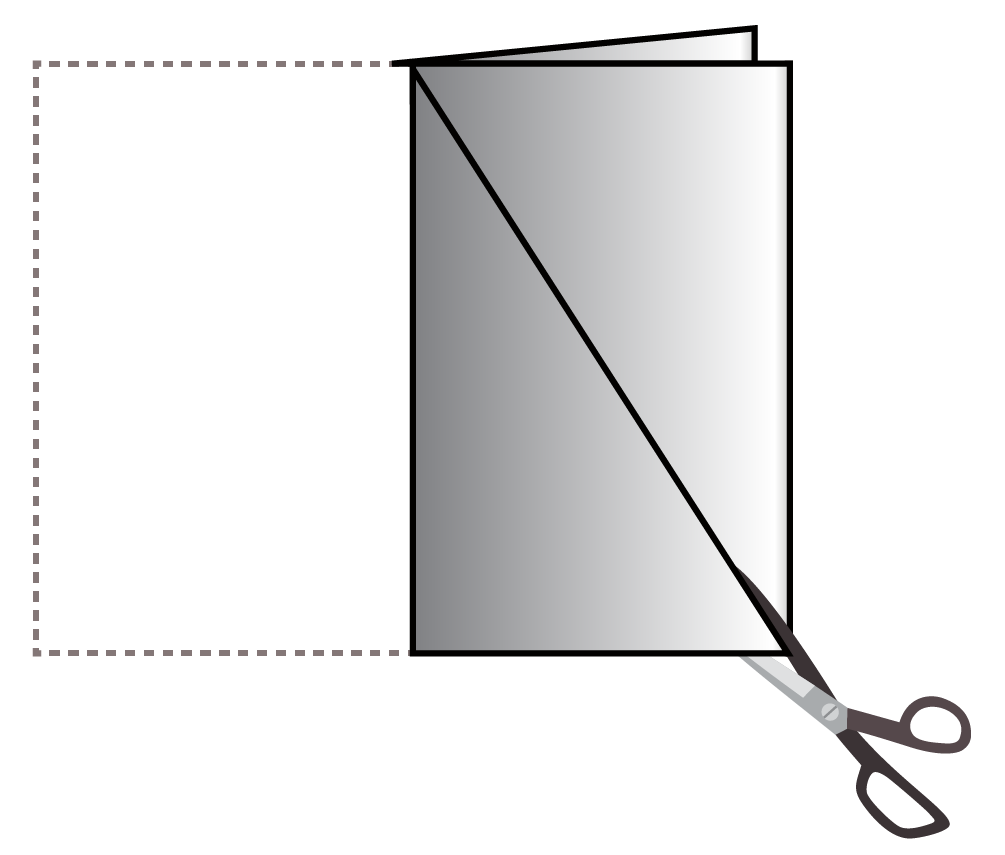You can see a triangle when you open the sheet.

Mark the vertices of the triangles as $$\text{A}$$, $$\text{B}$$, and $$\text{C}$$.

Now measure $$\text{AB}$$ and $$\text{AC}$$.

Repeat this activity with different measures and observe the pattern.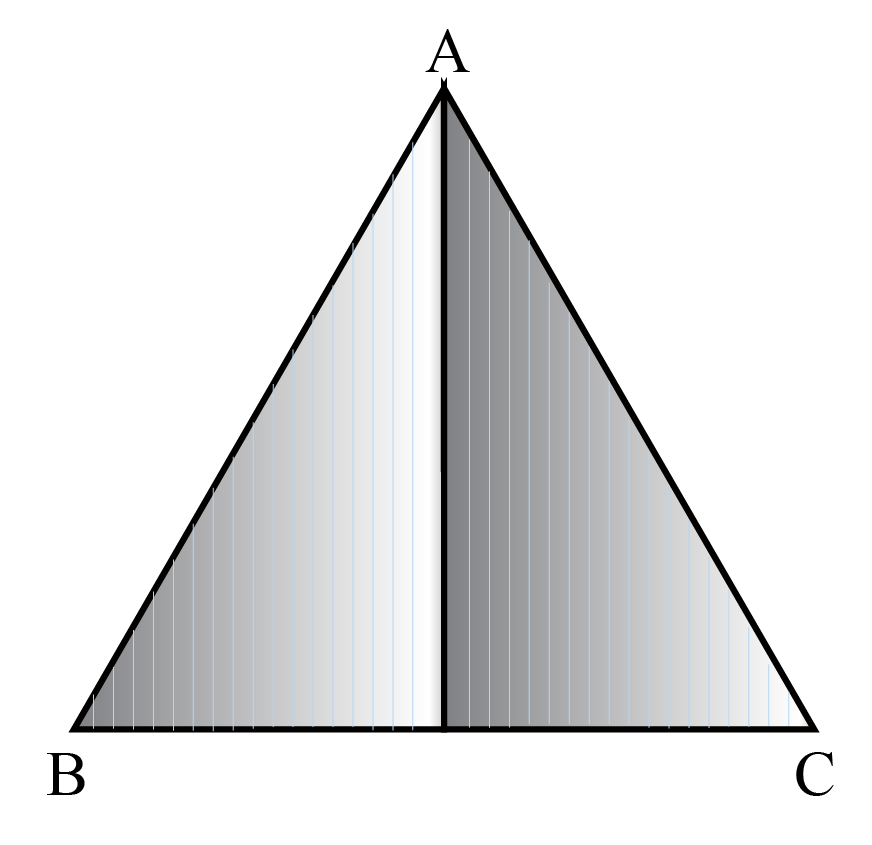We can observe that $$\text{AB}$$ and $$\text{AC}$$ are always equal.

This type of triangle where two sides are equal is called an isosceles triangle.

Here are a few isosceles triangle real-life examples.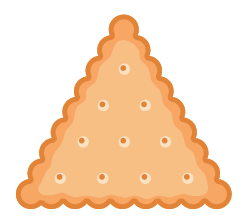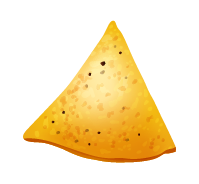## Isosceles Triangle Properties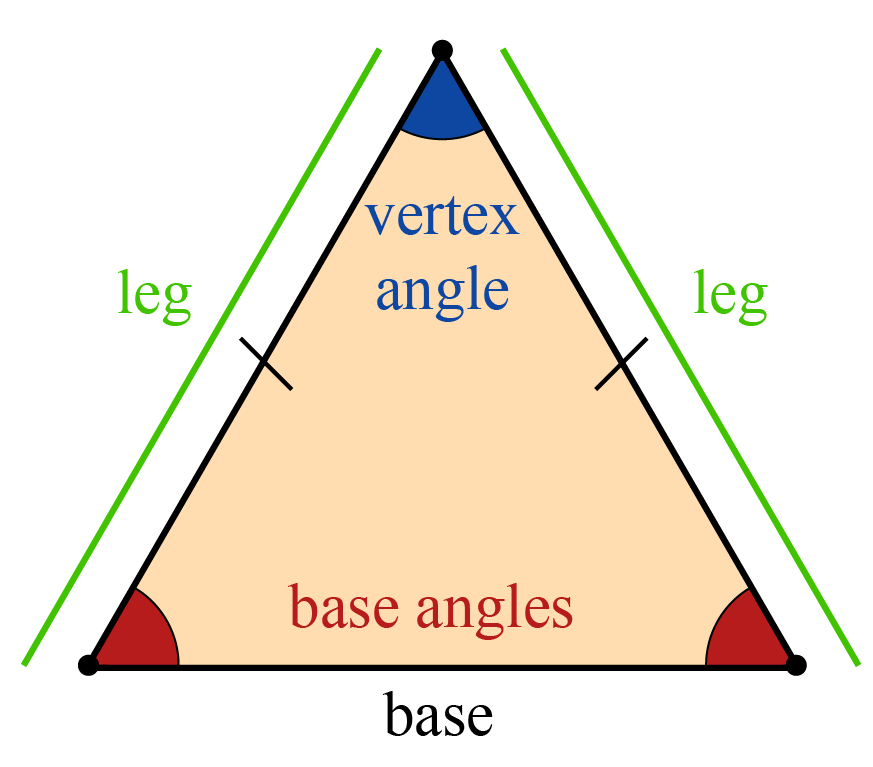• The two equal sides of an isosceles triangle are called the legs and the angle between them is called the vertex angle or apex angle.
• The side opposite the vertex angle is called the base and base angles are equal.
• The perpendicular from the vertex angle bisects the base and it also bisects the vertex angle.

## Isosceles Triangle Theorem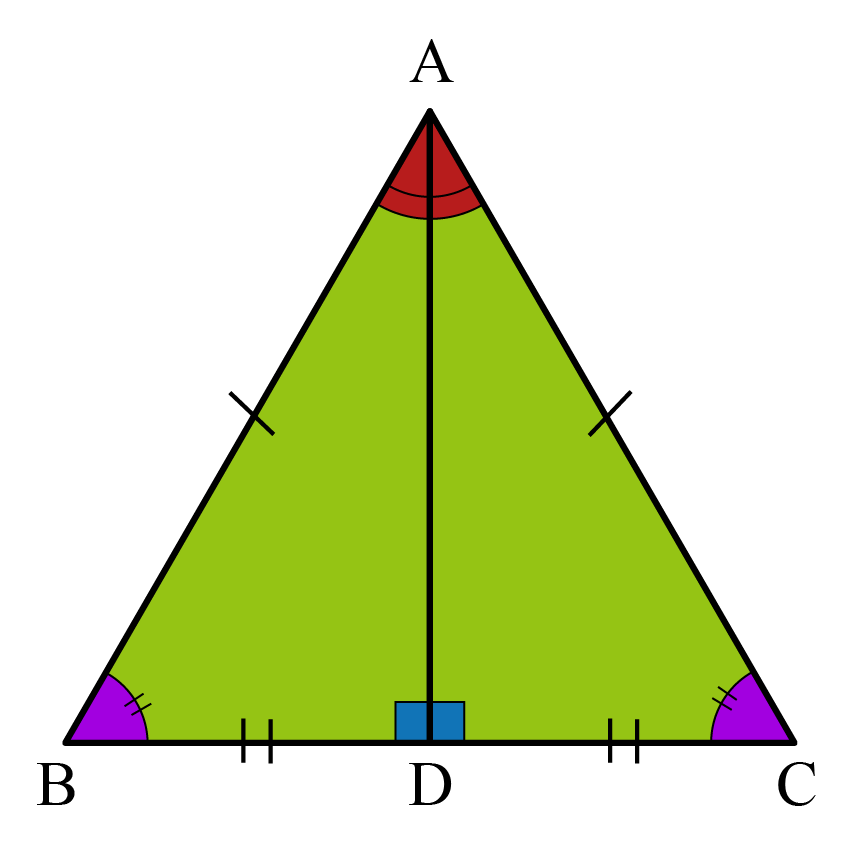• If two sides of a triangle are congruent, then angles opposite to those sides are congruent.
Conversely, if the two angles of a triangle are congruent, the corresponding sides are also congruent.

\begin{align} \angle \text{ABC} &= \angle \text{ACB} \\ \text{AB} &= \text{AC} \end{align}

• In an isosceles triangle, the altitude from the apex angle (perpendicular) bisects the base.
It also bisects the apex angle.

In the given triangle,

\begin{align} \angle \text{BAD} &= \angle \text{DAC} \\ \text{BD} &= \text{DC} \end{align}

Right triangles $$\Delta \text{ADB}$$ and $$\Delta \text{CDB}$$ are congruent.

## What is a Right Isosceles Triangle?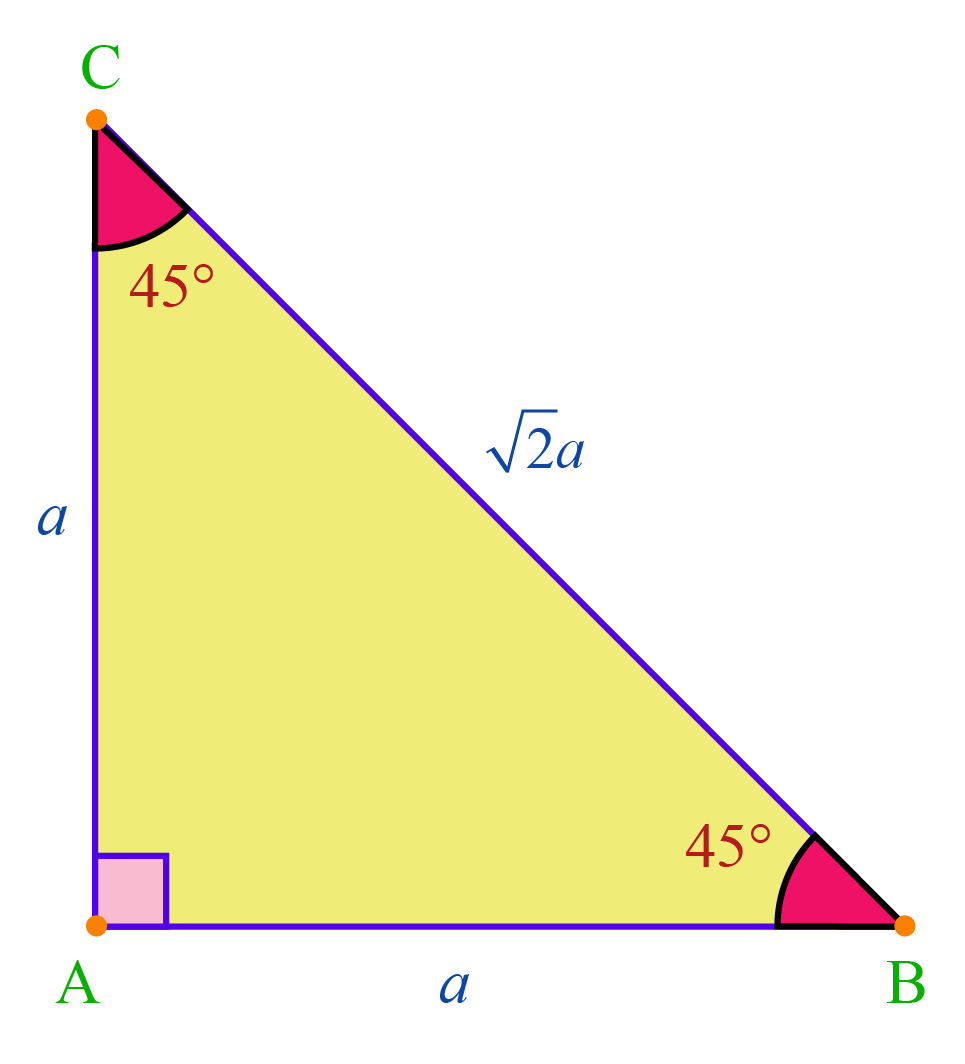In an isosceles triangle, if the vertex angle is $$90^\circ$$, the triangle is a right triangle.

A right isosceles triangle is a special triangle where the base angles are $$45 ^\circ$$ and the base is also the hypotenuse.

The hypotenuse of an isosceles right triangle with side $${a}$$ is

 $$\sqrt{2}a$$

## Isosceles Triangle Area Formula

The area of an isosceles triangle can be calculated in many ways based on the known elements of the isosceles triangle.

Known parameters Isosceles triangle formula
When the base $$b$$ and height $$h$$ are known  $\frac{1}{2} \times b \times h$
When all the sides $$a$$ and the base $$b$$ are known  $\frac{b}{2}\sqrt{\text{a}^2 - \frac{b^2}{4}}$
When the length of the two sides $$a$$ and $$b$$ and the angle between them $$\angle \text{α}$$ is known $\frac{1}{2} ab\:sin(\text{α})$

Use the calculator below to find the area of an isosceles triangle when the base and height are given.

Use the calculator below to find the area of an isosceles triangle when the base and the equal side are given.

Help your child score higher with Cuemath’s proprietary FREE Diagnostic Test. Get access to detailed reports, customized learning plans, and a FREE counseling session. Attempt the test now.

## Isosceles Triangle Solved Examples

 Example 1

In the given isosceles triangle $$\text{ABC}$$, find the measure of the vertex angle and base angles.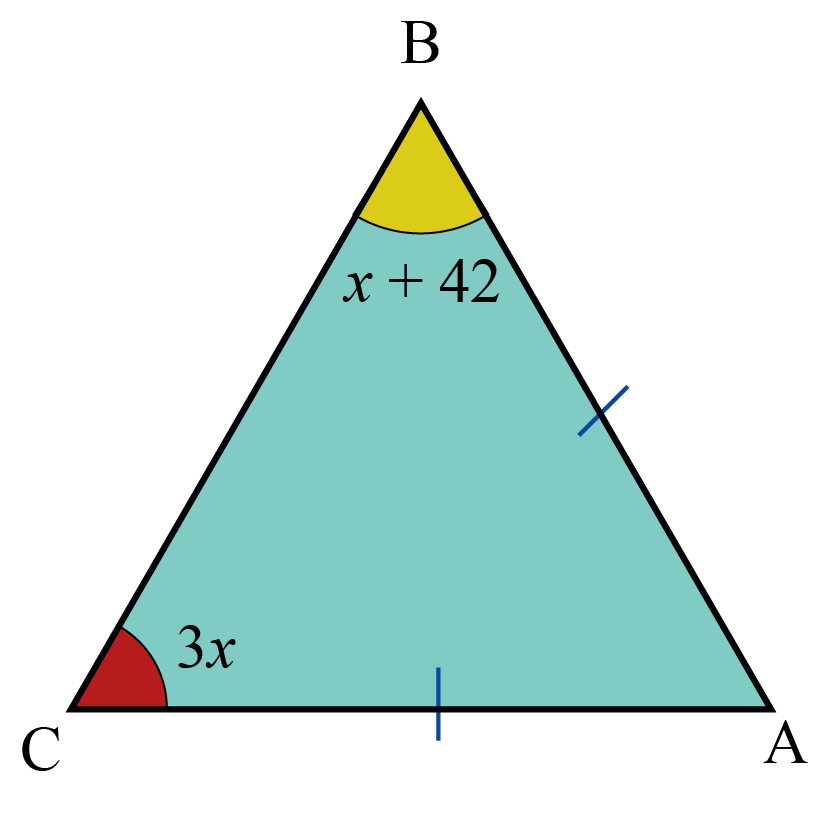Solution:

\begin{align} 3x &= x +42 (\because\angle \text{ABC} \! =\! \angle \text{BCA} )\\ \therefore 2x &= 42\\ x &=21\\ \angle \text{ABC} &= x+42\\ &= 63^\circ\\ \Rightarrow \angle \text{BCA} &=63^\circ(\!\because\!3x \!=\!3 \!\times\! 21\! =\!63\! )\\ \therefore \angle\text{BAC} &= (180-(63+63)\\ &=180-126\\ &=54^\circ \end{align}

 \begin{align}\angle \text{ABC}\!=\!\angle \text{BCA}\!=\!63^\circ \text{and} \:\angle\text{BAC}\!=\!54^\circ\end{align}
 Example 2

In the given figure, $$\text{AC = BC}$$ and $$\angle A = 30^\circ$$. What is the measure of $$\angle\text{ECD}$$?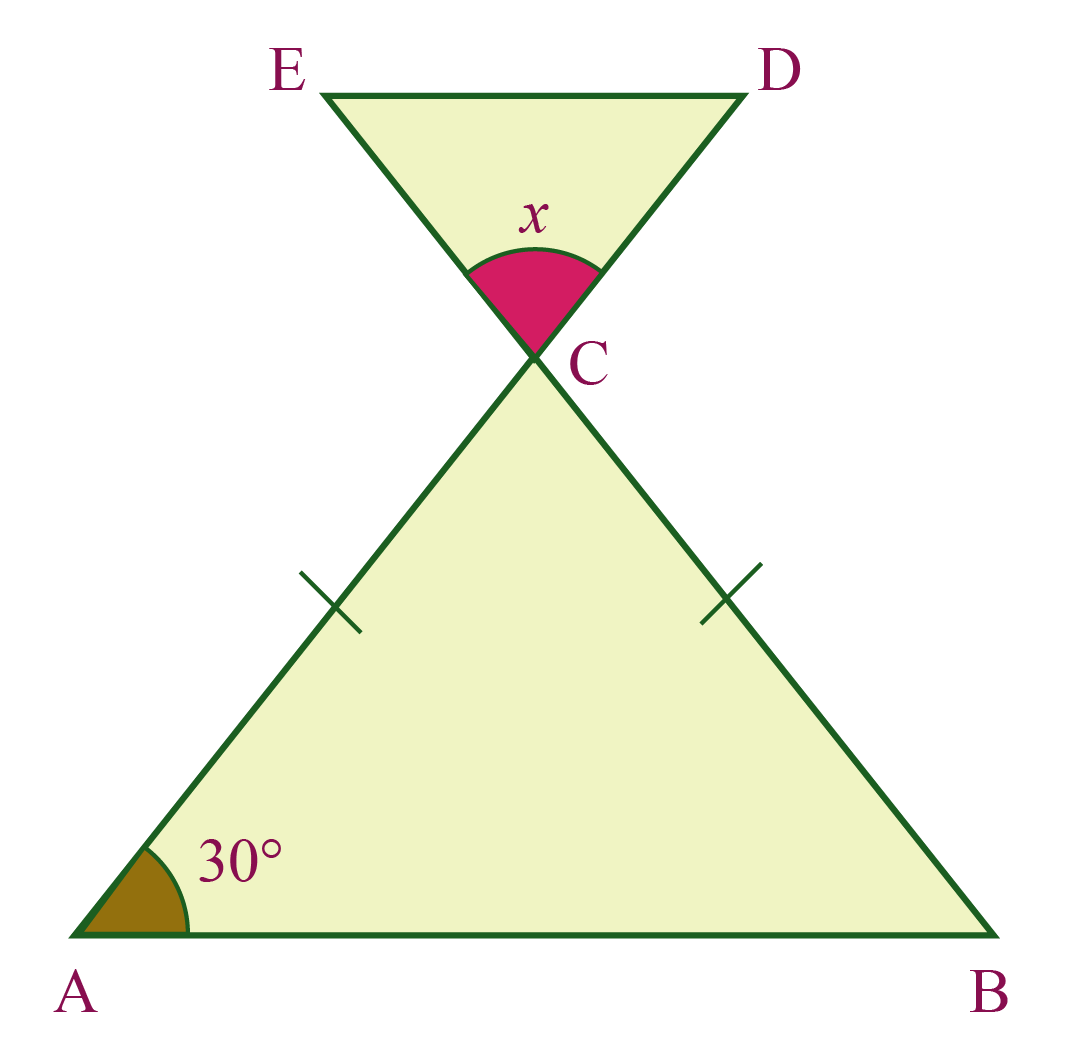Solution:

$$\Delta\text{ACB}$$ is isosceles as $$\text{AC = BC}$$

Therefore,

$$\angle A= \angle B$$

In $$\Delta\text{ACB}$$

\begin{align} \angle\text{CAB} +\angle\text{ABC}+\angle\text{BCA} &= 180^\circ\\ (\text{Sum of the angles of a triangle})\\ \Rightarrow \angle\text{BCA}\!&\!=\!180^\circ-(\!30^\circ\!+\!30^\circ) \\ \therefore \angle\text{BCA} &=120^\circ \\ \angle\text{BCA} &= \angle\text{DCE}\\ [\because \text{Vertically opposite angles are equal}]\\ \therefore x&=120^\circ \end{align}

 $$\therefore \angle \text{ECD} =120^\circ$$
 Example 3

In the given triangle, find the measure of BD and area of triangle ADB.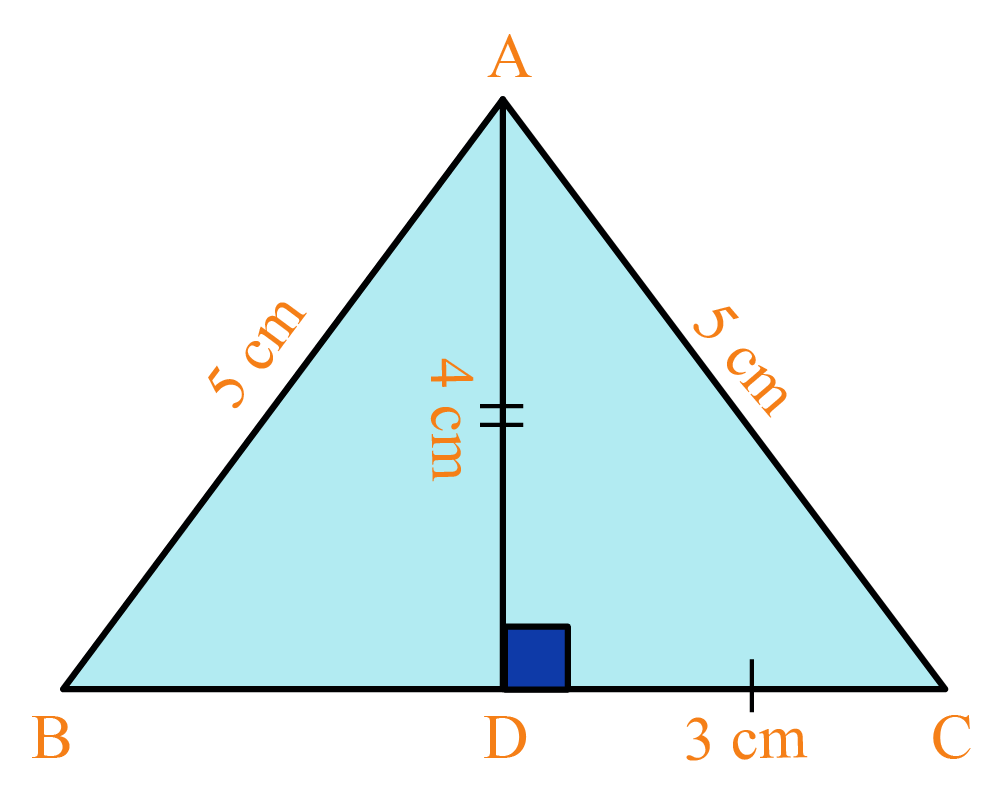Solution:

Given:

\begin{align} \text{AB} &= 5 \: \text{cm}\\ \text{AC} &= 5 \: \text{cm}\\ \text{AD} &= 4 \:\text{cm}\\ \text{DC} &= 3 \: \text{cm}\\ \text{AD}&\perp \text{BC} \end{align}

In an isosceles triangle, the perpendicular from the vertex angle bisects the base.

Therefore,

$$\text{BD} = \text{DC} = 3 \: \text{cm}$$

In $$\Delta \text{ADB}$$,

\begin{align} \text{Base}&=3\:\text{cm} \\ \text{Height}&=4\:\text{cm} (\text{given)}\\ \text{Area of} \Delta\text{ADC}&=\frac{1}{2}\times 3 \times 4 \\ &= 6\: \text{cm}^2 \end{align}

 $$\therefore \text{Area of } \Delta\text{ADB} = 6\: \text{cm}^2$$
 Example 4

In the given triangle $$\Delta \text{PQR}$$, find the measure of the perpendicular $$\text{QS}$$ (approx. to $$2$$ decimal places).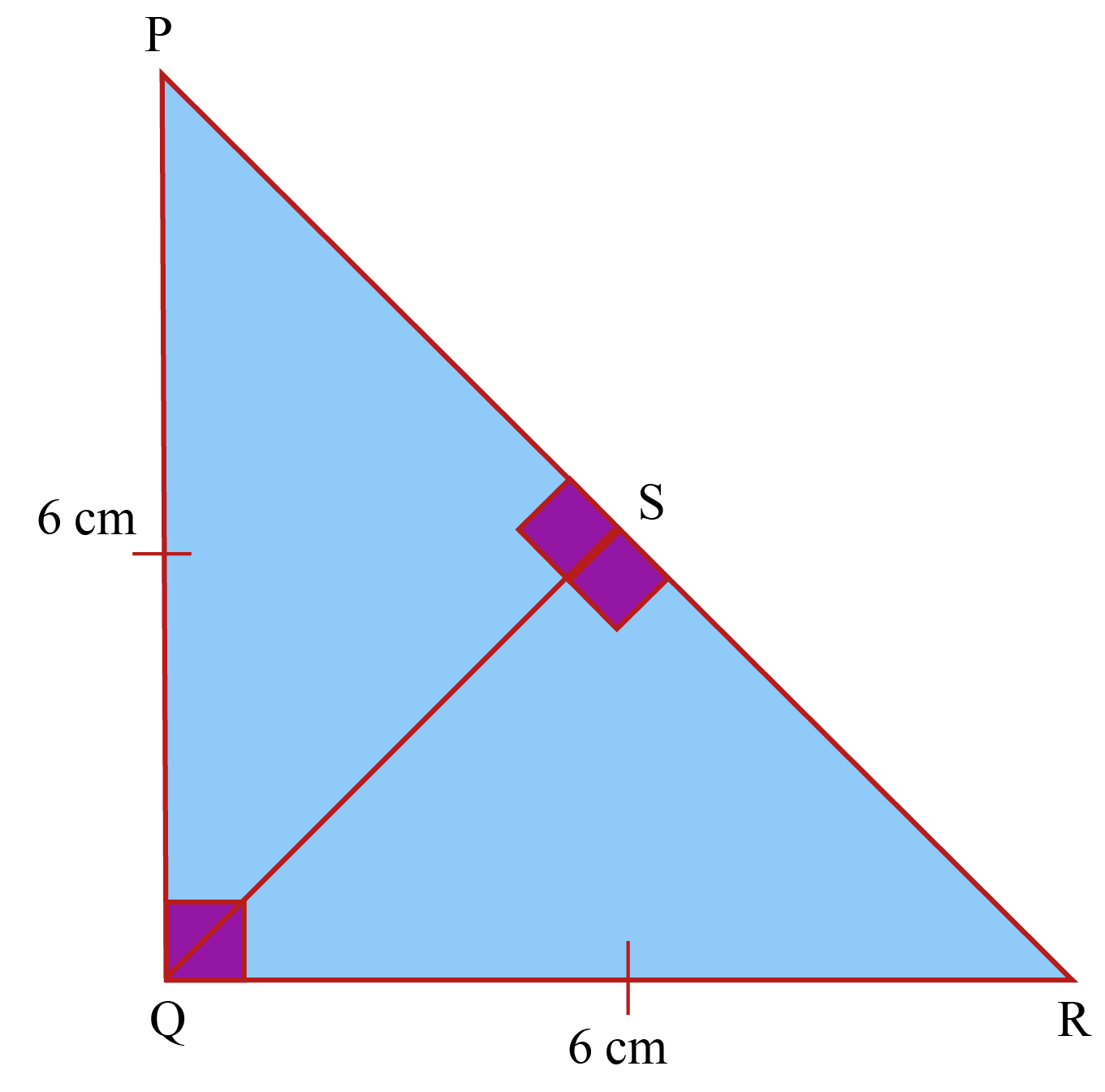Solution:

In the isosceles right triangle $$\Delta{PQR}$$, we have:

\begin{align} \text{PQ} &=6\: \text{cm} \\ \text{QR} &=6\: \text{cm} \\ \angle \text{PQR} &= 90^\circ \\ \text{QS} &\perp \text{PR} \end{align}

Area of $$\Delta{PQR}$$

\begin{align} &=\frac{1}{2} \times \text{Base} \times \text{Height} \\ &=\frac{1}{2} \times\text{PQ} \times \text{QR}\\ &=\frac{1}{2} \times 6 \times 6 \\ &=18 \:\text{cm}^2 \end{align}

Therefore, $$\text{PR}$$

\begin{align} &=6\sqrt{2} \: (\because \text{hypotenuse} = side\! \times\!\sqrt{2}) \\ &≈ 8.485\: \text{cm} \end{align}

Considering $$\text{PR}$$ as the base and $$QS$$ as the altitude, we have

\begin{align} \text{Area of }\Delta \text{PQR} &=\frac{1}{2} \times\text{Base} \times \text{Height} \\ \Rightarrow18 &=\frac{1}{2} \times\text{PR} \times \text{QS}\\ 18 &=\frac{1}{2} \times 8.485 \times\text{QS} \\ \therefore \text{QS} &= 4.24\: \text{cm} \end{align}

 $$\therefore \text{QS} = 4.24\: \text{cm}$$
 Example 5

Find the perimeter of an isoselese triangle, if the base is $$24\: \text{cm}$$ and the area is $$60 \:\text{cm}^2$$.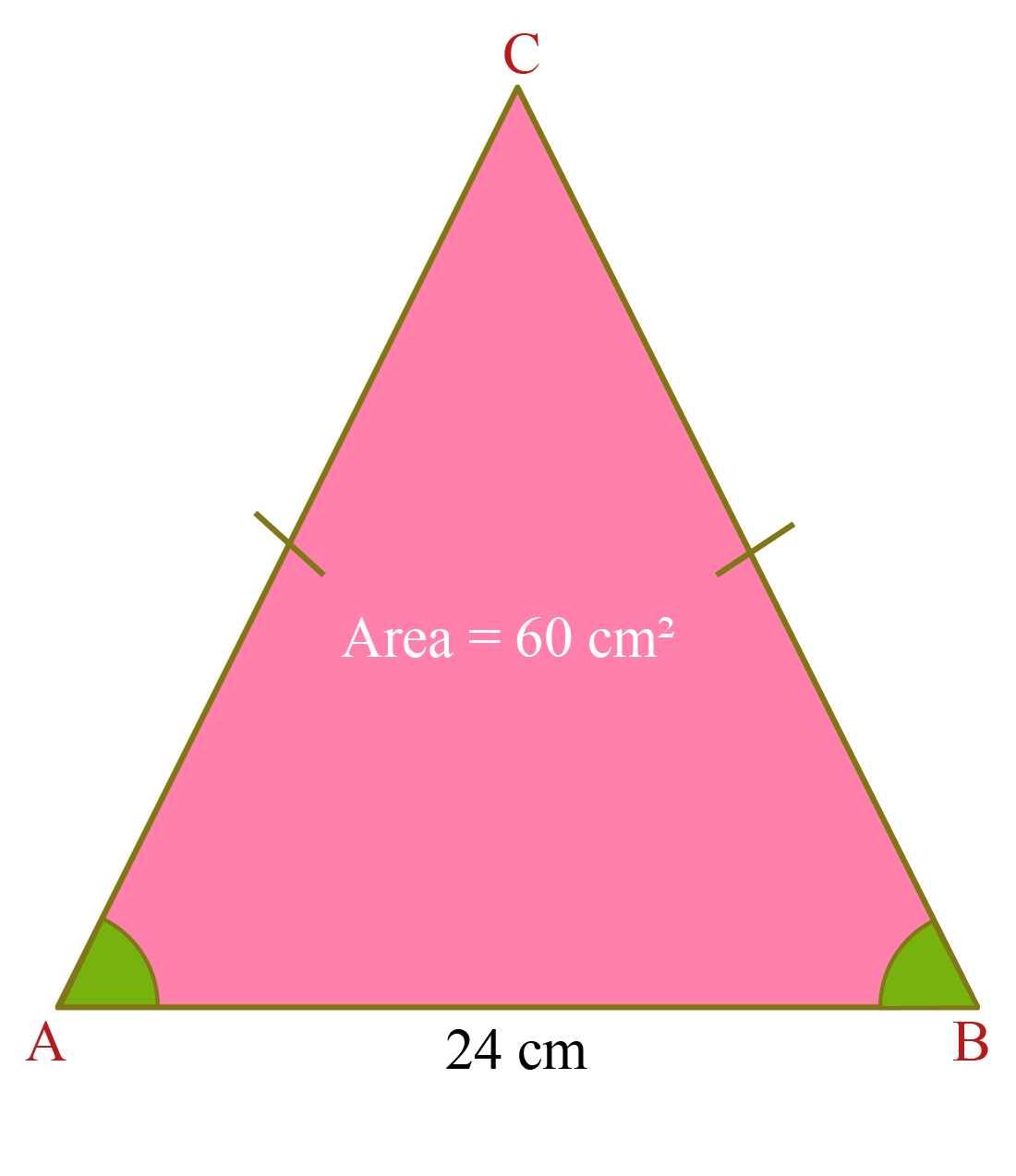Solution:

We know that,

\begin{align} \text{base} &= 24\: \text{cm}\\ \text{area} &=60 \:\text{cm}^2 \end{align}

Area of the triangle =

$$\frac{\text{b}}{2}\sqrt{\text{a}^2 - \frac{\text{b}^2}{4}}$$

Therefore,

\begin{align} \Rightarrow 60 &= \frac{24}{2}\sqrt{\text{a}^2 - \frac{24^2}{4}} \\ 60 &= 12\sqrt{\text{a}^2 - 144} \\ 5 &=\!\sqrt{\text{a}^2 \!-\!144} \: (\text{Squaring both sides}) \\ 25 &= \text{a}^2 -144 \\ \therefore \text{a}^2 &= 169 \\ \Rightarrow \text{a}&=13\: \text{cm} \end{align}

Perimeter of the isoselese triangle

\begin{align} &2\text{a}+\text{b} \\ &(2 \times 13) +24 \\ &=26+24 \\ &=50\: \text{cm} \end{align}

 $$\therefore$$ Perimeter of given triangle = $$50\: \text{cm}$$

CLUEless in Math? Check out how CUEMATH Teachers will explain Isosceles Triangles to your kid using interactive simulations & worksheets so they never have to memorise anything in Math again!

Explore Cuemath Live, Interactive & Personalised Online Classes to make your kid a Math Expert. Book a FREE trial class today!

## Practice Questions

Here are a few problems for you to practice. Select/Type your answer and click the "Check Answer" button to see the result.Challenging Questions
 1. In the given figure, PQ = QR and $$\angle \text{PQO} = \angle \text{RQO}$$. Prove that $$\angle \text{APQ} = \angle \text{BRQ}$$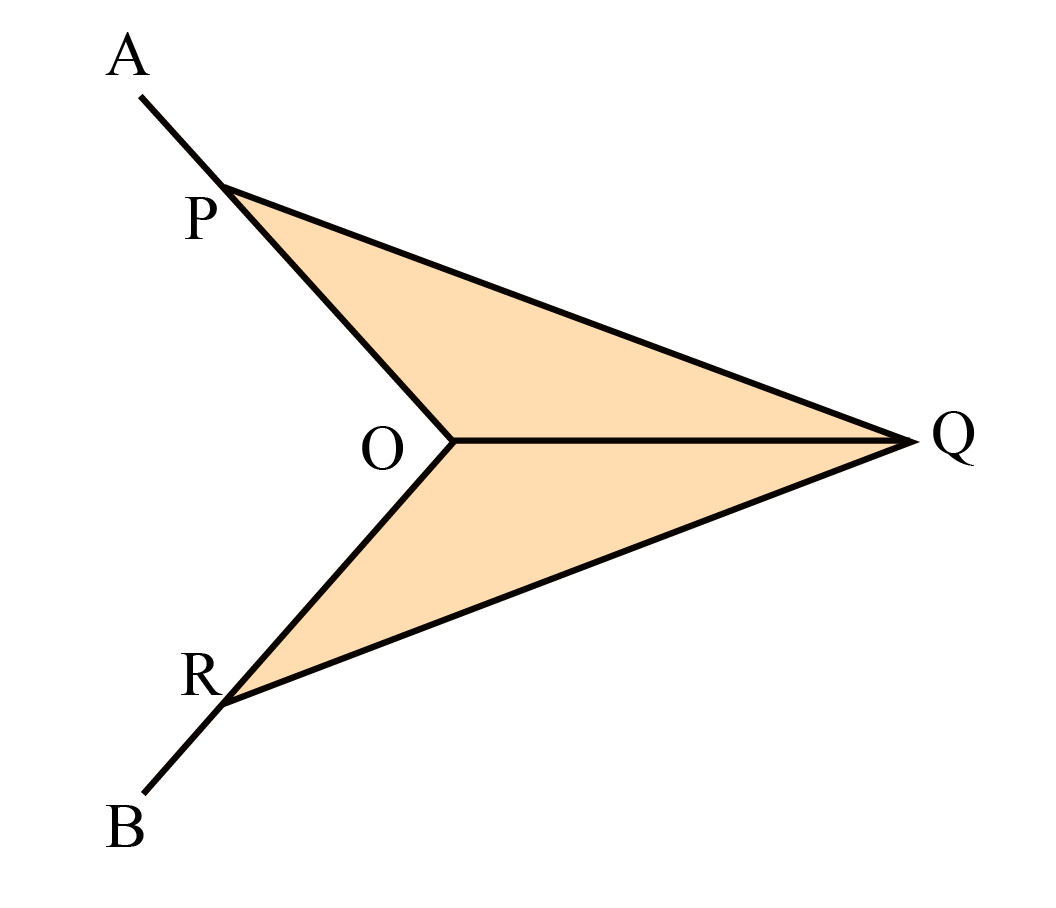2. Two sides of an isosceles triangle are 5 cm and 6 cm. What is the difference of the largest and the smallest possible perimeters?

IMO (International Maths Olympiad) is a competitive exam in Mathematics conducted annually for school students. It encourages children to develop their math solving skills from a competition perspective.

## 1. What is an isosceles triangle?

Isosceles triangle definition: A triangle in which two sides are equal is called an isosceles triangle.

## 2. What are the angles in an isosceles triangle?

• Vertex angle and the base angles are the angles in an isosceles triangle.
• The base angles measure the same.

## 3. Explain the isosceles triangle property.

• The isosceles triangle property states that when two sides are equal, the base angles are also equal.
• The perpendicular from the apex angle bisects the base.

grade 9 | Questions Set 2